### 3. Nomenclature & Parts

A single figure showing a spiraled horizontal curve with all its nomenclature, parts, and points labeled can be confusing. To make things a little more clear, we will use a series of figures emphasizing and explaining different attributes. Although ours will be a symmetric curve system, the same principles apply to differing entrance and exit spirals, as long as sufficient basic information is known.

Let's start with two tangents and a circular curve of radius Rc and length L connecting them, Figure E-6.Figure E-6 Simple curve between tangents

To make room for spirals inclusion requires pushing the circular arc in from the tangents and shortening its length. Adding two spirals, each Ls long, reduces the circular arc length to Lc. This results in the TS-SC-CS-ST curve system, Figure E-7. The OBC and OEC are offset positions of the BC and EC. Radial lines from the OBC and OEC to the arc's center of curvature are parallel with perpendicular lines at the TS and ST respectively.Ls: Spiral length Lc: Reduced arc length Rs: Spiral radius (varies) Rc: Circular arc radius TS: Tangent to Spiral SC: Spiral to Curve CS: Curve to Spiral ST: Spiral to Tangent OBC: Offset BC OEC: Offset EC Figure E-7 Spiraled horizontal curve

The radius of the entrance spiral, Rs, decreases uniformly from infinity at the TS to the circular arc radius, Rc, at the SC. The radius of the exit spiral increases uniformly from the arc radius at the CS to infinity at the ST.

The entrance spiral's degree of curvature, Ds, varies from 0°00'00" at the TS to the circular arc's, Dc, at the SC. Because it changes uniformly over the spiral's length, its rate is Equation E-1: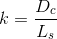Equation E-1 k: Spiral's Degree of Curvature rate change Dc: Circular arc D Ls: Spiral length

Although reversed, the exit spiral in a symmetric system has the same k.

Figure E-8 shows the central angles (Δ) for the spirals and circular arc.Δs: Spiral Central Angle Δc: Circular Curve Central Angle Figure E-8 Central Angles

Because the radial lines at the TS and OBC are parallel, the central angle of the entrance spiral is the same as the central arc angle from the OBC to the SC. The same is true for the exit spiral. The spiral angle is determined from Equation E-2.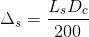Equation E-2

​The original circular arc's central angle is reduced by the spirals', Equation E-3.Equation E-3 Δc: Reduced arc central angle Δ: Total central angle Δs: Spiral central angle

And its shortened length is determined from Equation E-4 (modified from Chapter C. Horizontal Curves).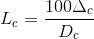Equation E-4

Location of the TS from the PI along with tangent distances and offsets from the TS to the OBC and SC are shown in Figure E-9.(a) (b) LCs: Spiral long chord A: Spiral deflection angle Ts: Spiral tangent Xo: Tangent distance to OBC o: Tangent offset to OBC  X: Tangent distance to SC  Y: Tangent offset to SC Figure E-9 Tangent Distances

The distances and offsets are computed using Equations E-5 through E-10Equation E-5Equation E-6Equation E-7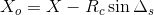Equation E-8Equation E-9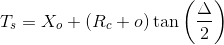Equation E-10

Because spirals are short and very flat, arc distances are often used to approximate chord distances. The is the case with Equations E-5 through E-7: the spiral length, Ls, is used for the spiral chord, LCs. In most cases, this assumption will be fine, however, we'll discuss a more exact, though more complex, spiral computing method also.